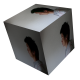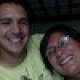Mapping qualities

Updated 2014 December 17th

Current high throughput sequencers produces reads that are short; for example the HiSeq2000 produces millions of reads that are 50 and 100 bp long. To align such short reads with high speed and accuracy, many short read alignment programs have been developed, such as BWA. The major limitation is the length of the sequenced reads because many eukaryotic genomes are repetitive and therefore it is difficult to accurately map these reads. Because of this, alignment programs have mapping qualities for each read that is mapped to the reference genome. A mapping quality is basically the probability that a read is aligned in the wrong place (i.e. phred-scaled posterior probability that the mapping position of this read is incorrect). The probability is calculated as:

$$!p = 10^{-q/10}$$

where q is the quality. For example a mapping quality of 40 = 10 to the power of -4, which is 0.0001, which means there is a 0.01 percent chance that the read is aligned incorrectly.

$$!p = 10^{-40/10} = 0.0001$$

Base calling errors with respect to mapping qualities

Sequencers make base calling mistakes and this complicates matters. To illustrate how this affects the mapping qualities using BWA, I will use an example I came across in SEQanswers. First let’s examine mapping qualities when a read maps to a specific region without suboptimal hits:

#what version of BWA

Program: bwa (alignment via Burrows-Wheeler transformation)
Version: 0.7.10-r789
Contact: Heng Li <lh3@sanger.ac.uk>

cat ref.fa
>ref
ACGTACGTACGTACGTACGTACGTAGGG
CCCCCCCCCCCCCCCCCCCCCCCCCCCC
CCCCCCCCCCCCCCCCCCCCCCCCCCCC
CCCCCCCCCCCCCCCCCCCCCCCCCCCC
CCCCCCCCCCCCCCCCCCCCCCCCCCCC
CCCCCCCCCCCCCCCCCCCCCCCCCCCC
CCCCCCCCCCCCCCCCCCCCCCCCCCCC

ACGTACGTACGTACGTACGTACGTA


Now to do the mapping:

bwa index ref.fa

#scroll to see the rest of the SAM file
@SQ	SN:ref	LN:196
read	0	ref	1	37	25M	*	0	0	ACGTACGTACGTACGTACGTACGTA	*	XT:A:U	NM:i:0	X0:i:1	X1:i:0	XM:i:0	XO:i:0	XG:i:0	MD:Z:25


Mapping the read to our reference, BWA returns a mapping quality of 37 (which is actually the highest mapping quality BWA returns).

Next let’s create an example with suboptimal hits. Below is a reference that contains five identical stretches of 28 mers and one 28 mer with a single mismatch (in red) compared to the other five:

>ref2
ACGTACGTACGTACGTACGTACGTAGGG
ACGTACGTACGTACGTAGGTACGTAGGG
ACGTACGTACGTACGTAGGTACGTAGGG
ACGTACGTACGTACGTAGGTACGTAGGG
ACGTACGTACGTACGTAGGTACGTAGGG
ACGTACGTACGTACGTAGGTACGTAGGG

Let’s map a read from the single mismatch stretch to this reference:

cat ref2.fa
>ref2
ACGTACGTACGTACGTACGTACGTAGGG
ACGTACGTACGTACGTAGGTACGTAGGG
ACGTACGTACGTACGTAGGTACGTAGGG
ACGTACGTACGTACGTAGGTACGTAGGG
ACGTACGTACGTACGTAGGTACGTAGGG
ACGTACGTACGTACGTAGGTACGTAGGG

ACGTACGTACGTACGTACGTACGTA

bwa index ref2.fa

#scroll to see the rest of the SAM file
@SQ	SN:ref2	LN:168
read2	0	ref2	1	16	25M	*	0	0	ACGTACGTACGTACGTACGTACGTA	*	XT:A:U	NM:i:0	X0:i:1	X1:i:5	XM:i:0	XO:i:0	XG:i:0	MD:Z:25


The mapping quality of the read in the second example is 16, which has a probability of $$10^{-16/10} = 0.025119$$ of mapping to the wrong place. Even though the read maps uniquely in the reference, its mapping quality is 16 and not 37. The BWA specific tags in the SAM file provides some nice additional information:

XT Type: Unique/Repeat/N/Mate-sw
X0 Number of best hits
X1 Number of suboptimal hits found by BWA

From the BWA tag information we can quickly deduce whether a read is aligned uniquely; in this case the XT:A:U indicates that it was aligned uniquely. In addition, the X1:i:5 tag indicates that there were 5 suboptimal hits.

Mapping qualities when considering base calling errors

To model base calling errors we can use the Binomial distribution; if I expect there to be 1 base calling error in 100 bps, I can calculate the probability of an error for a read of 25 nt as such using R

#Probability of success (1 error in 100 bases) = 0.99
#Number of trials (each base is a trial) = 25

#no base calling errors, i.e. 25 successes
dbinom(x = 25, size=25, prob=0.99)
# 0.7778214

#one base calling error, i.e. 24 successes
dbinom(x = 24, size=25, prob=0.99)
# 0.1964195

#two base calling errors, i.e. 23 successes
dbinom(x = 23, size=25, prob=0.99)
# 0.02380843


If we expect 1 base calling error in 100 bps, the probability of making two base calling errors in 25 bps is quite low. Using the formula from the SEQanswers post that calculates the posterior probability that the best alignment is actually correct:

$$! \frac{P_{zero\ base\ errors}}{P_{zero\ bases\ errors} + 5 \times P_{one\ base\ error}}$$

Calculating this in R:

#the posterior probability that the best alignment is correct
p = 0.99
dbinom(x = 25, size=25, prob=p) / (dbinom(x = 25, size=25, prob=p) + (5 * dbinom(x = 24, size=25, prob=p)))
# 0.4419643


In reality base calling is much more accurate than 1 error in 100 bases, which is a Phred quality score of 20. If we changed the base calling error rate to 1 in 1000 (Phred score of 30):

p = 0.999
dbinom(x = 25, size=25, prob=p) / (dbinom(x = 25, size=25, prob=p) + (5 * dbinom(x = 24, size=25, prob=p)))
# 0.88879


then the posterior probability that the best alignment is correct improves to 0.88879. Using a base calling error rate of 1 in 10000 (Phred score of 40):

p = 0.9999
dbinom(x = 25, size=25, prob=p) / (dbinom(x = 25, size=25, prob=p) + (5 * dbinom(x = 24, size=25, prob=p)))
# 0.9876531


improves the probability to 0.9876531, which is a ~0.012 probability that the alignment is incorrect, which is around the same ball park to the BWA mapping quality of 16, which is a 0.025 probability that the alignment is incorrect.

Does BWA make use of base calling qualities?

When I included base calling qualities to the read

ACGTACGTACGTACGTACGTACGTA
+
!!!!!!!!!!!!!!!!!!!!!!!!!

I still get the same mapping quality of 16 with BWA, indicating that mapping qualities are not used by BWA:

tag 0 artificial 1 16 25M * 0 0 ACGTACGTACGTACGTACGTACGTA !!!!!!!!!!!!!!!!!!!!!!!!! XT:A:U NM:i:0 X0:i:1 X1:i:5 XM:i:0 XO:i:0 XG:i:0 MD:Z:25

This was confirmed when I examined the BWA manual, which mentioned that “Base quality is NOT considered in evaluating hits.”.
1.Saad says:

I recently emailed Heng li author of bwa. Since in the new bwa another program called bwa-mem is introduced and is recommended by the author of the software to use with longer reads >75 bp.

Bwa-mem does not seem to produce XT,XM and XO tags I was wondering what would would be the efficient way to get the optimal number of uniquely mapping reads. According to Heng li it is to use mapping quality but I have seen posts at seqanswers and biostars who say that using mapping quality “q1” through samtools would not give correct results. So my question is what value of MAPQ should be used to get the optimal number of uniquely mapping reads.

regards

1.Davo says:

Thanks for the comment and for the information; I haven’t tried BWA-MEM yet.

I don’t know about BWA-MEM, but I know some programs that use a mapping quality of 3 to indicate a read mapping to two places. This is because 10^(-3/10) = 0.5011872. A mapping quality of 1 indicates that a read maps to five places. If that is the case, then a mapping quality of 10 gives a 90% probability that the read is mapped correctly. I don’t know what an “optimal number” is, but q10 seems to be “reasonable”.

Hope that helps,

Dave

2.Juan Montenegro says: